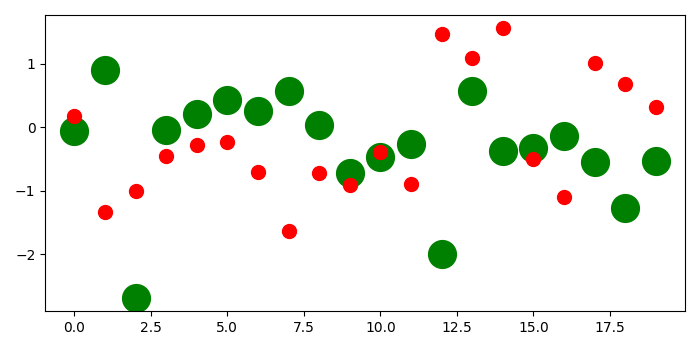# Plot scatter points using plot method in Matplotlib

To plot scatter points using plot method in matplotlib, we can take the following steps−

• Create random data points (x1 and x2) using numpy.
• Plot x1 data points using plot() method with marker size 20 and green color.
• Plot x2 data points using plot() method with marker size 10 and red color.

## Example

import numpy as np
from matplotlib import pyplot as plt
plt.rcParams["figure.figsize"] = [7.00, 3.50]
plt.rcParams["figure.autolayout"] = True
x1 = np.random.randn(20)
x2 = np.random.randn(20)
plt.plot(x1, 'go', markersize=20)
plt.plot(x2, 'ro', ms=10)
plt.show()

## Output